# Newton’s Second Law

## Rotational Motion of Class 11

Since torque is a rotational analog of force, therefore, Newton’s second law for rotational motion is given by

τnet = Iα (10.21)

Note that the above equation (10.21) is not a vector equation.

It is valid in two situations:

(i)The axis is fixed in position and direction.

(ii)The axis passes through the center of mass and is fixed in direction only.

The equation τcm = Icmαcm (10.22)

is valid even if the center of mass is accelerating.

Example 10.8

A disc-shaped pulley has mass M = 4 kg and radius R = 0.5 m. It rotates freely on a horizontal axis, as in figure (10.14). A block of mass m = 2 kg hangs by a string that is tightly wrapped around the pulley.

(a)What is the angular velocity of the pulley 3 s after the block is released?

(b)Find the speed of the block after it has fallen 1.6m. Assume the system starts at rest.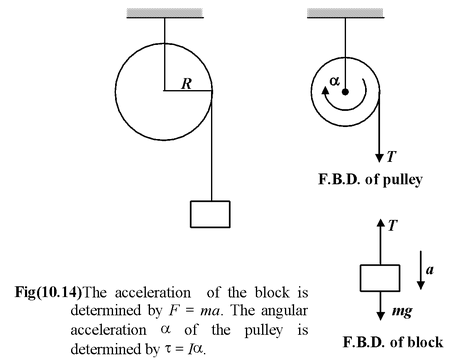Solution

Since the string is tangential to the pulley, the torque on it due to the tension is τ = TR. The two forms of Newton's second law for the block and the pulley yield

Block(F = ma)mg - T = ma

Pulley (τ = Iα)TR =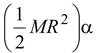Applying Newton’s second Law

τ = Iα

For the disc, I = 1/2MR2

∴TR =orT =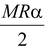(i)

Applying Newton’s second Law on the block

Fnet = ma

∴mg – T = ma(ii)

Since the block and the rim of the pulley have the same speed (the string does not slip), we have v = ωR and a = αR. Thus, from equation (i) we find

T = 1/2 Ma   (iii)

a =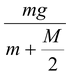(iv)

Putting m = 2 kg; M = 4 kg; R = 0.5m;

we get a = 5 m/s2

(a)To find ω after 3 s, we use equation (10.9)

ω = ωo + αt = 0 + (a/R)t = 30 rad/s

(b)To find the speed of the block we use

v2 = vo2 + 2ay = 0 + 2(5 m/s2)(1.6 m)

Thusv = 4 m/s

 Example 10.9 For the arrangement shown in the figure (10.15), the string is slightly wrapped over the pulley. Find the acceleration of each block when released from rest. The string is not slipping over the pulley.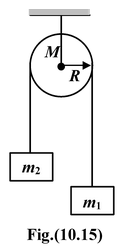Solution

 The free body diagrams of the pulley and the blocks are shown in the figure. Note that tensions on two sides of the pulley are different. Why? Applying Newton’s second law on the pulley, we get τ = T1R – T2R = (T1 – T2)R Sinceτ = Iα =Therefore, (T1 – T2)R =orT1 – T2 =(i) Applying Newton’s Law on the blocks, we get T2 – m2g = m2a2 (ii) m1g – T1 = m1a1(iii)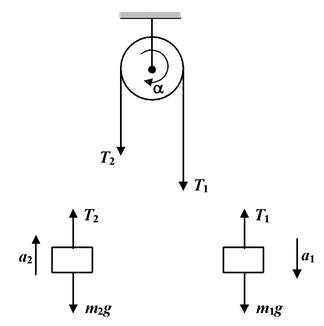Since the string is tightly wrapped over the pulley, therefore,

a1 = a2 = αR = a (iv)

Solving equations (i), (ii), (iii) and (iv), we obtain

a =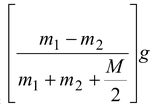Example 10.10 A fly wheel of mass M = 2 kg and radius R = 40 cm rotates freely at 600 rpm. Its moment of inertia is 1/2MR2. A brake applies a force F = 10 N radially inward at the edge as shown in the figure. If the coefficient of friction is μk = 0.5, how many revolutions does the wheel make before coming to rest?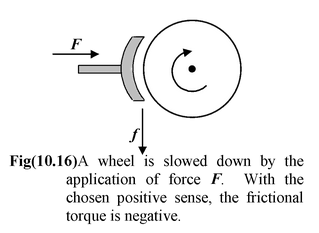Solution

We choose the initial sense of the angular velocity as positive. The force of friction is
f = μkF and its (counterclockwise) torque is τ = -fR.

Using τ = Iα, we have

-(μkF)R =orα =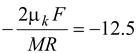rad/s2

The angular rotation θ of the wheel is given by

ω2 = ωo2 + 2αθ

Hereωo = 2πN/60 = 2π(600)/60 = 20π rad/s

Example 10.11

 A uniform rod of length L and mass M is pivoted freely at one end as shown in the figure (10.17). (a)What is the angular acceleration of the rod when it is at angle θ to the vertical? (b)What is the tangential linear acceleration of the free end when the rod is horizontal? The moment of inertia of the rod about one end is 1/3ML2.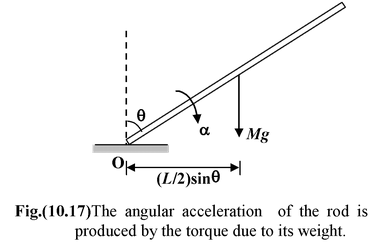Solution

Figure (10.17) shows the rod at an angle θ to the vertical.

Net torque about the point O is

τo = Mg L/2 sin θ

Using II law of motion

τo = Ioα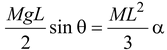Thus,

α = 3g sin θ/2L

(b)When the rod is horizontal θ = π/2 and α = 3g/2L. From equation (10.13), the tangential linear acceleration is

at = αL = 3g/2

This is greater than the acceleration of an object in free-fall.